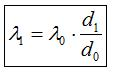23. ANGLE SHIFT  PART I       THE CHANGE OF THE SIGNAL EMISSION ANGLEIf the arrival target of a signal is a reference system that is in motion, depending on the direction of the movement of the target, the signal emission angle changes. I named this event Angle Shift.In the figure above, when the plane is at (x1,y1,z1) coordinates, lets discuss a signal that the station on the ground emits and that targets the plane as its arrival point. The signal sets out at t1 moment when the plane is at (x1,y1,z1) coordinates. During the travel time of the signal, the plane continues its movement in its own direction of movement. In the end, the signal reaches the plane at t2 moment and at (x2,y2,z2) point.If the plane wasnt in motion, the same signal would reach the plane at (x1,y1,z1) point. However, as the plane is in motion, the signal reaches the plane at (x2,y2,z2) point. To make it easy to think about it, lets think of a single photon. While the signal (photon) would normally go to (x1,y1,z1) point, it goes to (x2,y2,z2) point instead. For such a situation to occur, it is necessary for the signal to change its direction during its emission, which it does indeed; it changes its direction.The direction change of the signal occurs in parallel with signal wavelength change and at the moment of signal emission. The reason the signal changes its direction is the same. Whatever reason leads to signal wavelength change, the same reason also determines the direction of the movement of the signal. In the figure, the angle where d1 and d3 lines intersect gives us the amount of change in the angle of the movement of the signal relative to the reference system of the transmitter.It is going to be a kind of repetition, but Im writing it again: d3 line in the figure is the direction of the outgoing signal relative to the reference system of the signal tower. d1 line in the figure is the direction of the incoming signal relative to the reference system of the plane (Remember that we assume the plane is motionless and the signal station is in motion.).There is no rule saying that Angle Shift occurs all the time. If the movements of the source and target reference systems are on the same line (if it happens on a single dimension), the signal emission angle doesnt change. In all cases except this, Angle Shift occurs.We saw that wavelength change can be described based on distances.d0 : The distance that the INCOMING signal covers relative to the receiver  d1: The distance that the OUTGOING signal covers relative to the transmitter λ0 : Wavelength of the transmitters factory setting λ1 : Wavelength measured at the receiverIf we pay attention, for a wavelength change to occur, the lengths of d0 and d1 distances must be different from each other; this situation by itself shows that Angle Shift is a must.In the figure, we see a plane that is coming towards the signal tower from the skyline in the direction of the Z-axis. The red line shows the arrival route of the plane. If we pay attention, relative to the reference system of the signal tower, while the position of the plane on the Z-axis decreases, its position on the Y-axis consistently increases. Lets divide the movement of the signal into small Doppler Triangles relative to the signal tower. Assume that, when the plane is at point A (x0,y6,z6), a signal that sets out from the signal tower reaches the plane at point B (x0,y5,z5). Also suppose that, after this, when the plane is at point B (x0,y5,z5), a signal that set out from the signal tower reaches the plane at point C (x0,y4,z4). As can be seen, for the example in the figure, Angle Shift occurs in such a way that the angle increases. If the movement of the plane was on the same line and in the direction of going away, Angle Shift would occur in a way that the angle decreases.Angle Shift is a dynamic variable. It is always in a state of change depending on speeds, directions, and locations of reference systems, and, during the emission of the signal, it is a value specific to that moment and that signal.Angle Shift is a crucial effect that is worthy of notice. It is a special result that is peculiar to Alice Law. No other theory except Alice Law has the chance to show the existence of this situation. Angle Shift is one of the most important arguments that establish and lead the future of physics and direct theoretical physics. The fact that it is an effect that can be identified empirically further increases its importance.Ill keep discussing Angle Shift in Chapter Three.